Next: 3. Thermoelectric Properties of Graphene-Based Nanostructures Up: 2.4 Phonon Transport Previous: 2.4.1 Landauer Formula   Contents

## 2.4.2 Boltzmann Transport Equation

At equilibrium, the distribution of phonons in branchand wavevector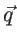is given by the Bose-Einstein distribution function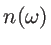: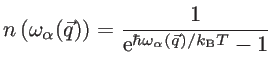(2.59)

Under non-equilibrium conditions, the distribution of phonons deviates from its equilibrium distribution, and transport of phonons is computed using the Boltzmann transport formalism. The non-equilibrium distribution function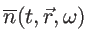, in general, is a function of time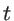and position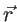. The BTE can be written as: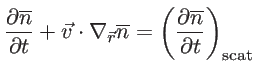(2.60)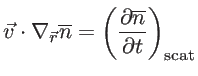(2.61)

Under a temperature gradient, the BTE can be written as :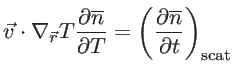(2.62)

In the relaxation time approximation, the change of the distribution function due to the scattering events can be given by: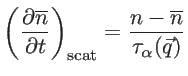(2.63)

and therefore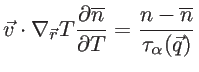(2.64)

where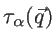is the relaxation time of phonons of frequency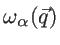. In this work we use a linearized form of Eq. 2.64, which assumes that the temperature gradient causes only a small deviation from Bose-Einstein distribution function [61,62], so that: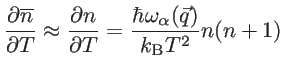(2.65)

and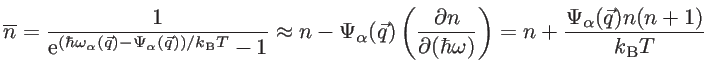(2.66)

where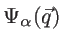shows the deviation from the equilibrium distribution. Then, one may eliminate the temperature gradient using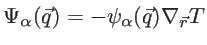and write: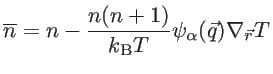(2.67)

Since the equilibrium distribution does not carry any heat flux, the heat flux equals to :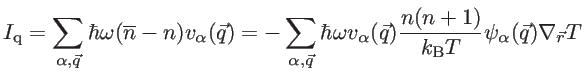(2.68)

On the other hand, it holds the differential form of Fourier's law: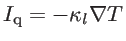(2.69)

Therefore, one can obtain the lattice thermal conductivity as: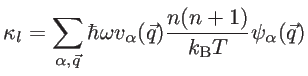(2.70)

Under the single-mode relaxation time (SMRT) approximation ,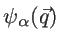follows from the linearized BTE (Eqs. 2.64-2.66) as: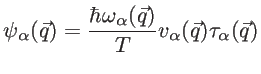(2.71)

Here,is the scattering time in SMRT approximation. Therefore, Eq. 2.70 becomes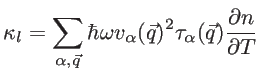(2.72)Next: 3. Thermoelectric Properties of Graphene-Based Nanostructures Up: 2.4 Phonon Transport Previous: 2.4.1 Landauer Formula   Contents
H. Karamitaheri: Thermal and Thermoelectric Properties of Nanostructures